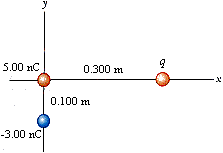# Problem: Three point charges are arranged as shown in Figure. (a) Find the vector electric field that the q = 7.50 nC and -3.00 nC charges together create at the origin. N/C  +      N/C (b) Find the vector force on the 5.00 nC charge.

###### FREE Expert Solution

Electric field:

$\overline{){\mathbf{E}}{\mathbf{=}}\frac{\mathbf{k}\mathbf{q}}{{\mathbf{r}}^{\mathbf{2}}}}$

Coulomb's force:

$\overline{){\mathbf{F}}{\mathbf{=}}{\mathbf{q}}{\mathbf{E}}}$

(a)

k = 9.0 × 109 N•m2/C2

Ex = (9.0 × 109 × 7.50 × 10-9)/0.3002 = 750 N/C

Ey = (9.0 × 109 × -3.00 × 10-9)/0.1002 = - 2700 N/C

82% (323 ratings)###### Problem Details

Three point charges are arranged as shown in Figure.(a) Find the vector electric field that the q = 7.50 nC and -3.00 nC charges together create at the origin.

N/C+      N/C(b) Find the vector force on the 5.00 nC charge.